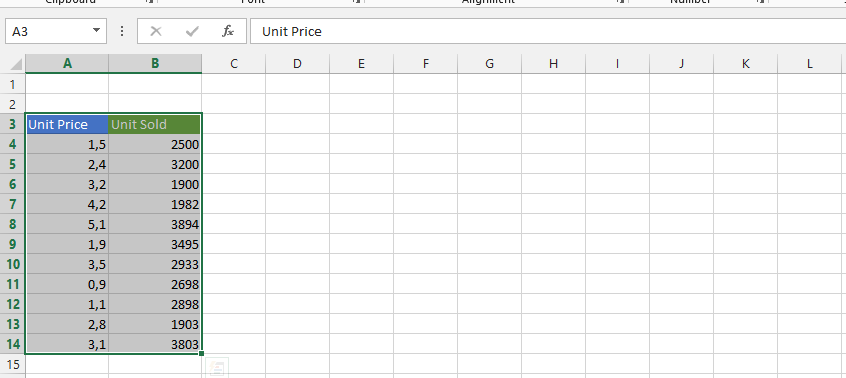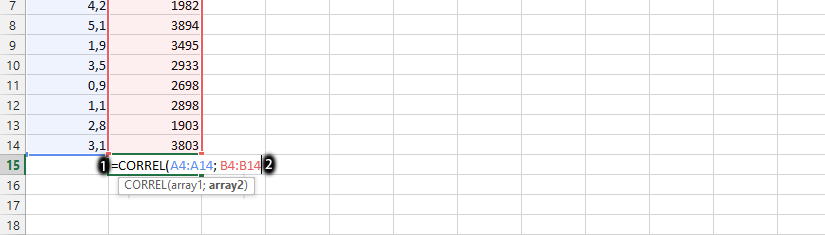#### How to Calculate Correlation in Excel

The correlation coefficient is a statistical measure that represents the strength and direction of the relationship between two variables. A correlation coefficient of 1 indicates a perfect positive correlation, meaning that as one variable increases, the other variable increases as well. A correlation coefficient of -1 indicates a perfect negative correlation, meaning that as one variable increases, the other variable decreases. A correlation coefficient of 0 indicates no correlation.

In Microsoft Excel, you can calculate the correlation coefficient using the CORREL function. This article will show you how to calculate the correlation coefficient in Excel and how to interpret the results.

## Step 1: Gather Your Data

To calculate the correlation coefficient, you will need two columns of data that are related to each other. For example, you could use the unit price and quantity of sold units to determine if there is any correlation between them.## Step 2: Enter the Formula

Once you have gathered your data, you can enter the formula for the correlation coefficient in Excel.

The formula is as follows: =CORREL(range1, range2)

• range1 is the first range of data that you want to correlate.
• range2 is the second range of data that you want to correlate.

For example, if your data is in cells A1:A14 and B1:B14, you would use the following formula:

=CORREL(A4:A14,B4:B14)## Step 3: Interpret the Results

The correlation coefficient will be a number between -1 and 1. A value of 1 indicates a perfect positive correlation, meaning that as one variable increases, the other variable increases as well. A value of -1 indicates a perfect negative correlation, meaning that as one variable increases, the other variable decreases. A value of 0 indicates no correlation.

For example, if the correlation coefficient is 0.7, this means that there is a strong positive correlation between the two variables. This means that as the unit price increases, the quantity of sold units also tends to increase.

Conclusion

Calculating the correlation coefficient in Excel is simple and straightforward using the CORREL function. By analyzing the correlation coefficient, you can determine the strength and direction of the relationship between two variables, and use this information to make informed decisions or predictions.

## Key Takeaways

The correlation coefficient is a statistical measure that represents the strength and direction of the relationship between two variables.

The correlation coefficient can be calculated in Excel using the `CORREL` function.

The correlation coefficient can be interpreted as follows:

• A value of 1 indicates a perfect positive correlation, meaning that as one variable increases, the other variable increases as well.
• A value of -1 indicates a perfect negative correlation, meaning that as one variable increases, the other variable decreases.
• A value of 0 indicates no correlation.

## FAQ

Q: What is the difference between correlation and causation?

A: Correlation does not equal causation. This means that just because two variables are correlated does not mean that one causes the other. For example, there may be a correlation between the number of ice cream sales and the number of drownings, but this does not mean that ice cream causes drownings.

Q: What are the limitations of the correlation coefficient?

A: The correlation coefficient is only a measure of the strength and direction of the relationship between two variables. It does not tell you anything about the cause of the relationship. Additionally, the correlation coefficient can be affected by outliers, so it is important to check your data for outliers before calculating the correlation coefficient.